# Mathematics Support

Math 6 | Unit 1 2 3 4 5 6 7 8 9

### Area and Surface Area

Reasoning to Find Area

Parallelograms

Triangles

Polygons

Surface Area

## Reasoning to Find Area

Before grade 6, your student learned to measure the area of a shape by finding the number of unit squares that cover the shape without gaps or overlaps. For example, the orange and blue shapes each have an area of 8 square units.In grade 6, students learn to find the areas of more complicated shapes using two ideas:

• Two shapes that “match up exactly” have the same area. For example, triangles A and B have the same area because Triangle A can be placed on Triangle B so they match up exactly.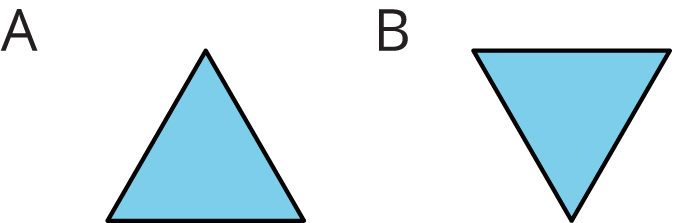• We can decompose (break) a shape into smaller pieces and find its area by adding the areas of the pieces. For example, the area of the shape on the left is equal to the area of Rectangle A, plus the area of Rectangle B, plus the area of Rectangle C.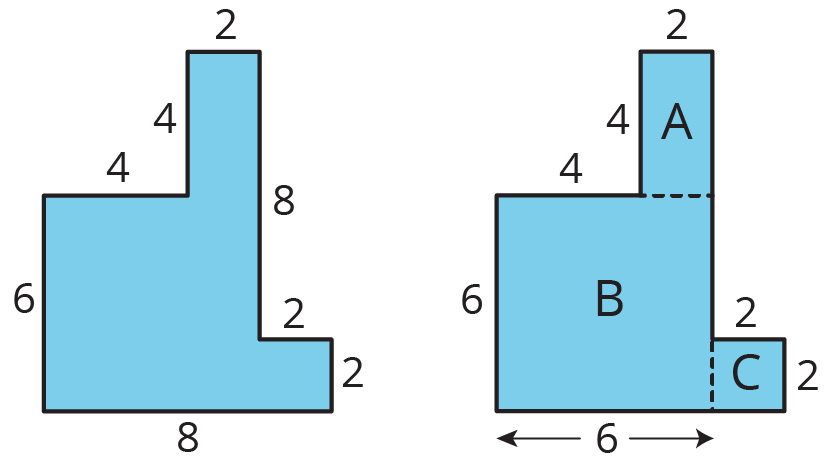It is sometimes helpful to rearrange the pieces of a shape in order to find its area. For example, the rectangular piece that is 2 units by 4 units at the top of the shape can be broken and rearranged to make a simple rectangle that is 8 units and 6 units. We can easily find the area of this rectangle (48 square units, because 8×6=48).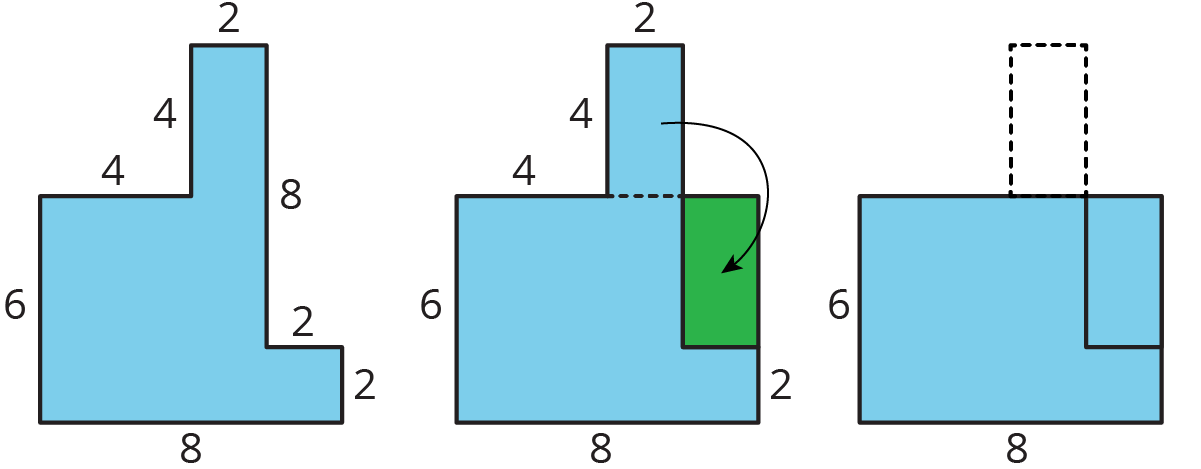The area of the square is 1 square unit. Find the area of the entire shaded region. Show your reasoning.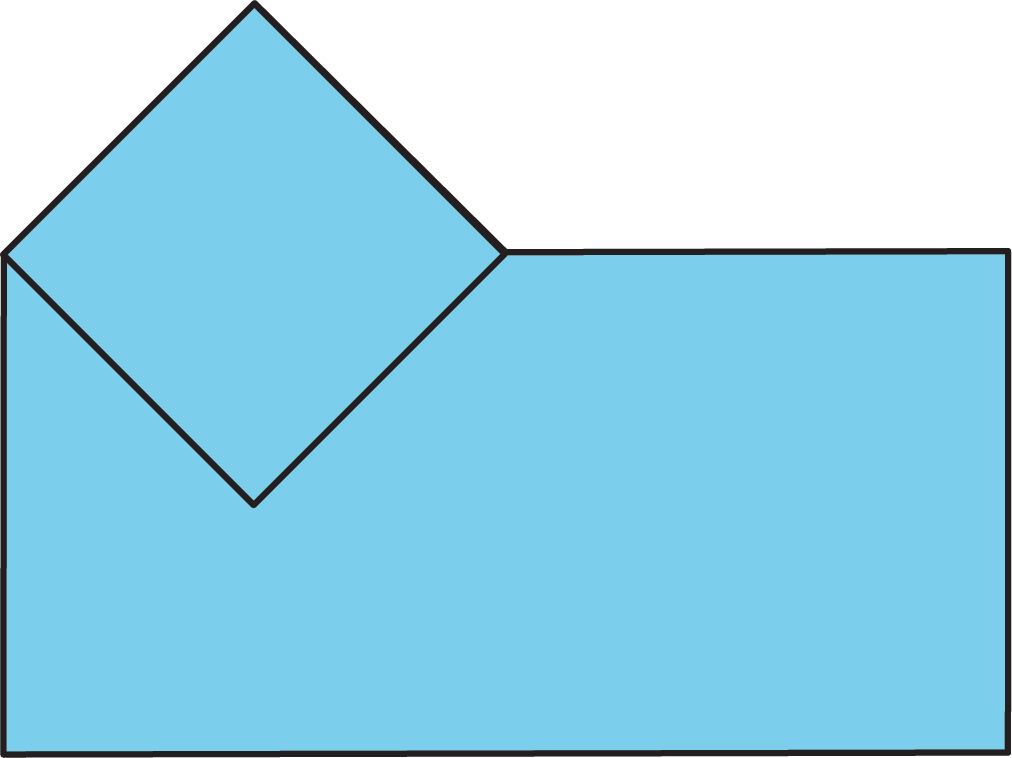Solution:

41/square units. Sample reasoning: The rest of the region can be decomposed into a square and several triangles. Two triangles can be arranged to match up perfectly with a square, so each triangle has half the area of the square (12 square units). In the entire shape, there is a total of 2 squares (2 square units) and 5 triangles (5×12 or 2+12 square units). 2 + 212= 412.## Parallelograms

This week, your student will investigate parallelograms, which are four-sided figures whose opposite sides are parallel.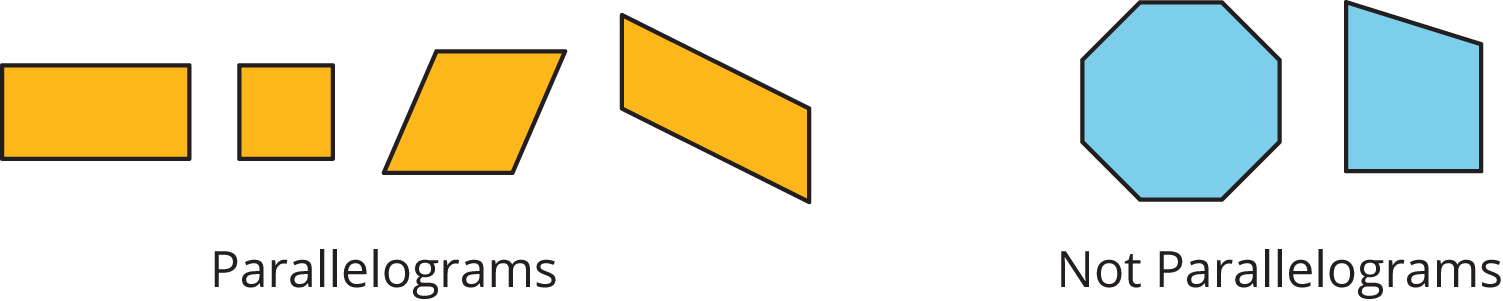We can find the area of a parallelogram by breaking it apart and rearranging the pieces to form a rectangle. The diagram shows a few ways of rearranging pieces of a parallelogram. In each one, the result is a rectangle that is 4 units by 3 units, so its area is 12 square units. The area of the original parallelogram is also 12 square units.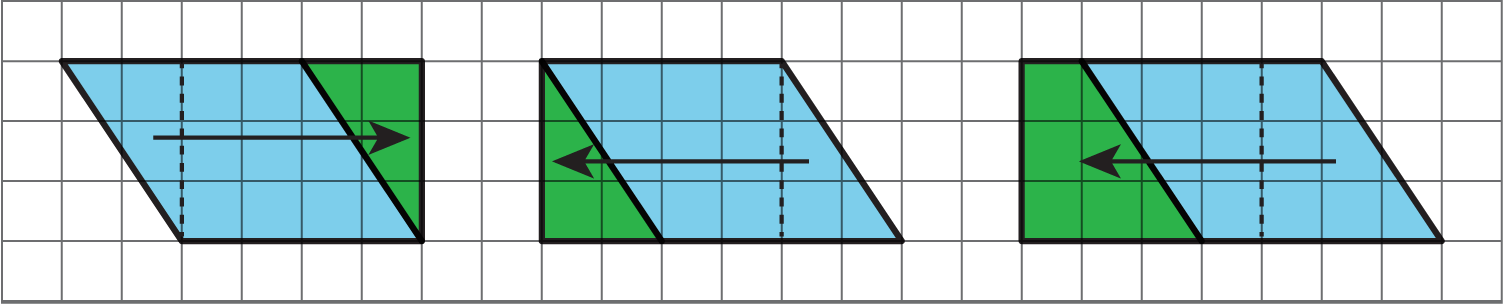Using these strategies allows students to notice pairs of measurements that are helpful for finding the area of any parallelogram: a base and a corresponding height. The length of any side of a parallelogram can be used as a base. The height is the distance from the base to the opposite side, measured at a right angle. In the parallelogram shown here, we can say that the horizontal side that is 4 units long is the base and the vertical segment that is 3 units is the height that corresponds to that base.

The area of any parallelogram is base×height.

Elena and Noah are investigating this parallelogram.Elena says, “If the side that is 9 units is the base, the height is 7.2 units. If the side that is 7.5 units is the base, the corresponding height is 6 units.”

Noah says, “I think if the base is 9 units, the corresponding height is 6 units. If the base is 7.5 units, the corresponding height is 7.2 units.”

Do you agree with either one of them? Explain your reasoning.

Solution:

Agree with Noah. Explanations vary. Sample explanation: A corresponding height must be perpendicular (drawn at a right angle) to the side chosen as the base. The dashed segment that is 6 units is perpendicular to the two parallel sides that are 9 units long. The dashed segment that is 7.2 units long is perpendicular to the two sides that are 7.5 units.

## Triangles

Your student will now use their knowledge of the area of parallelograms to find the area of triangles. For example, to find the area of the blue triangle on the left, we can make a copy of it, rotate the copy, and use the two triangles to make a parallelogram.This parallelogram has a base of 6 units, a height of 3 units, and an area of 18 square units. So the area of each triangle is half of 18 square units, which is 9 square units.

A triangle also has bases and corresponding heights. Any side of a triangle can be a base. Its corresponding height is the distance from the side chosen as the base to the opposite corner, measured at a right angle. In this example, the side that is 6 units long is the base and the height is 3 units.

Because two copies of a triangle can always be arranged to make a parallelogram, the area of a triangle is always half of the area of a parallelogram with the same pair of base and height. We can use this formula to find the area of any triangle:

12×base×height

Find the area of each triangle. Show your reasoning.

1.1.Solution:

1. 12 square feet. Sample reasoning: The triangle is half of a rectangle that is 3 feet by 8 feet, which has an area of 24 square feet.
2. 152 square units. Sample reasoning: The triangle is half of a parallelogram with a base of 5 units and a height of 3 units. 1253=152.

## Polygons

Knowing how to find the area of triangles allows your student to find the area of polygons, which are two-dimensional shapes made up of line segments. The line segments meet one another only at their end points. Triangles, quadrilaterals, pentagons, hexagons, etc., are all polygons.

To find the area of any polygon, we can break it apart into rectangles and triangles. Here is a polygon with 7 sides and one way to break it apart into triangles. Finding the areas of all triangles and adding them gives the area of the original polygon.Find the area of polygons A and B. Explain or show your reasoning.Solution:

A: 12 square units, B: 18 square units. Sample diagram and explanations:Polygon A can be broken into two triangles. The one on the left has base 6 units and height 3 units, so its area is 9 square units (1263=12). The one on the right has base 6 units and height 1 unit, so its area is 3 square units (1261=3). The total area is 9+3 or 12 square units.

Polygon B can be broken into a rectangle and two triangles. The area of the top triangle is 1241 or 2 square units. The rectangle is 8 square units. The area of the bottom triangle is 1244 or 8 square units. 2+8+8=18

## Surface Area

Imagine painting all of the sides of a box. The amount of surface to be covered with paint is the surface area of the box. Your student will focus on finding the surface areas of different three-dimensional objects such as the prisms and pyramids shown here.

One way to find the surface area of a three-dimensional object is to draw its net, which shows all the faces of the object as a two-dimensional drawing. A net can be cut out and folded to make the object. To find the surface area of the object, we can find the area of each face (as shown on the net) and add them. The areas of the six rectangular faces shown add up to 76 square units because 10+20+10+20+8+8=76, so the surface area of this box is 76 square units.Andre drew a net of a triangular prism and calculated its surface area. He made an error in both the net drawing and in the calculation.1. Identify Andre’s errors.
2. Find the correct surface area for the prism. Show your reasoning.

Solution:

Net: The triangles in a triangular prism should be identical, but the net shows two different triangles. Calculation: There are a few errors. The area of each triangle should be 1283 or 12 square units. Andre did not multiply the base and height by half. The wrong calculation is repeated for both triangles. In the calculation for the surface area, Andre doubled the area of the largest rectangle (which is 16 square units) while there is only one rectangle with that area.
The surface area should be 60 square units. The combined area of the two triangles should be 2(1283) or 24 square units. 10+10+16+24=60. Sample corrected net:IM 6–8 Math was originally developed by Open Up Resources and authored by Illustrative Mathematics, and is copyright 2017-2019 by Open Up Resources. It is licensed under the Creative Commons Attribution 4.0 International License (CC BY 4.0). OUR's 6–8 Math Curriculum is available at https://openupresources.org/math-curriculum/.

Disclaimer: This site provides external links and videos as a convenience and for informational purposes only. The appearance of external hyperlinks on the MCPS Family Mathematics Support Center website does not constitute an endorsement by the Montgomery County Public School System of any of the products or opinions contained therein.